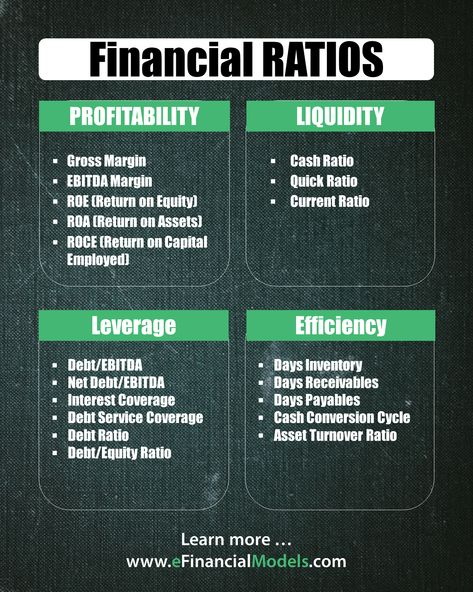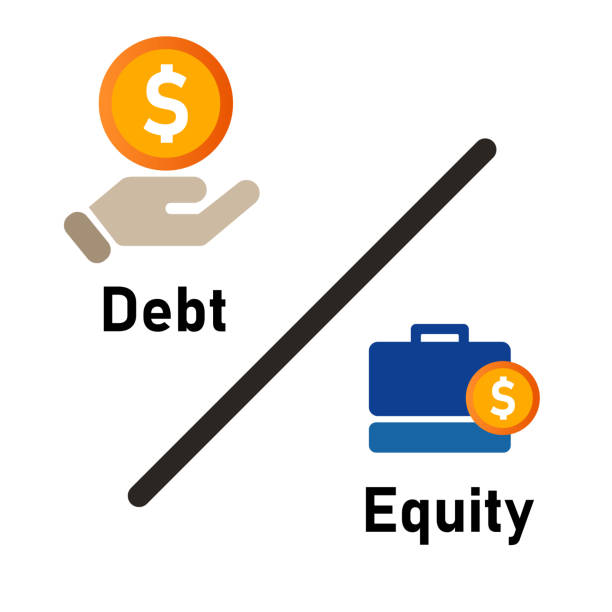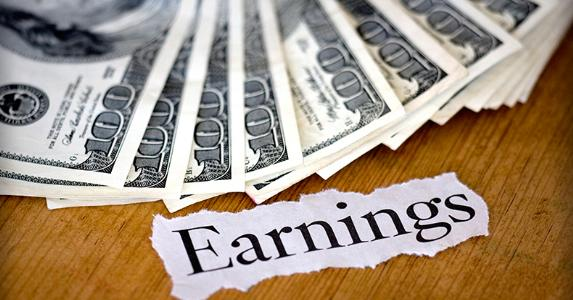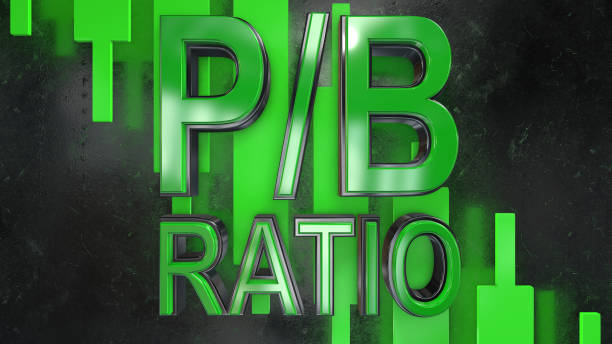# Financial Ratios

Made with the utilization of mathematical qualities taken from budget reports

Financial ratios are made with the utilization of mathematical qualities taken from budget reports to acquire significant data about an organization.The numbers found on an organization's financial reports - balance sheet, cash flow statement, and income statements - are utilized to perform the quantitative examination and evaluate an organization's liquidity, leverage, development, margins, profitability, rates of return, and valuation.

Uses of Financial ratios

Financial ratios have the following uses:

1. For Making Comparisons

One of the purposes of financial ratio analysis is to compare an organization's financial performance with comparable firms in the sector to grasp the organization's situation on the lookout.

Obtaining financial ratios, like Price/Earnings, from known contenders and comparing them with the organization's proportions can help executives distinguish market holes and analyze its competitive advantages and disadvantages.

The administration can then utilize the data to form choices that can advance the organization's position in the market.

2. For identifying trends

Organizations can also utilize financial ratios to check whether there is a trend in financial figures. Large organizations gather information from financial reports over a large number of reporting periods.

The pattern received can be utilized to anticipate the course of future financial performance and recognize any normal monetary disturbance that wouldn't be imaginable to foresee involving proportions for a single revealing period.3. For Determining Operational Efficiency

The administration of an organization can likewise utilize financial ratio analysis to decide the level of effectiveness in the administration of assets and liabilities.

Wasteful utilization of assets like automobiles, land, and buildings brings about superfluous costs that should be disposed of. Financial ratios can likewise assist with deciding whether the monetary assets are finished or under-utilized.

4. For Determining the performance of a company

Determining individual financial ratios per period and following the adjustment of their values over the long run is done to recognize patterns that might be created in an organization.

For instance, a rising debt-to-asset ratio might show that an organization is overburdened with debt and may ultimately be confronting default risk.

## Categories of Financial Ratios

1. Liquidity Ratios

Liquidity ratios measure an organization's capacity to meet its debt commitments utilizing its current assets.

Nearing the point when an organization is encountering financial hardships and can't pay its debts, it can change over its assets into money and utilize the cash to settle any forthcoming debts without any difficulty.

Some commonly used liquidity ratios are the quick ratio, the cash ratio, and the current ratio. Liquidity ratios are utilized by banks, lenders, and providers to decide whether a client can respect their monetary commitments.

2. Solvency Ratios

Solvency ratios measure an organization's long-term financial feasibility. These ratios look at the debt levels of an organization to its assets, equity, or yearly profits.

Significant solvency ratios are- debt to capital ratio, debt ratio, interest conversion ratio, and equity multiplier. Solvency ratios are predominantly utilized by state-run administrations, banks, employees, and institutional financial backers.

3. Profitability Ratios

Profitability ratios measure a business's capacity to earn profits compared with its related costs. Recording a higher profitability ratio than in the previous financial year's reporting shows that the business is improving financially.

A profitability ratio can also be compared to a comparative company's ratio to decide how profitable the business is compared with its rivals.

Some of the significant profitability ratios are the return on equity ratio, return on assets, profit margin, gross margin, and return on capital employed.

4. Efficiency Ratios

Efficiency ratios measure how well the business is utilizing its assets and liabilities to create deals and earn profits. They compute the utilization of inventory, machinery utilization, and turnover of liabilities, as well as the use of equity.

These ratios are significant because when there is an improvement in the efficiency ratios, the business can produce more income and profits.

Efficiency ratios include the asset turnover ratio, inventory turnover, payables turnover, working capital turnover, fixed resource turnover, and receivables turnover ratio.

5. Coverage Ratios

Coverage ratios measure a business' capacity to support its debts and different commitments. Analysts utilize the coverage ratios across regular reporting periods to draw a pattern that predicts the organization's future financial position.

A higher coverage ratio implies that a business can support its debt and related commitments effortlessly.

Key coverage ratios are the debt coverage ratio, interest coverage, fixed charge coverage, and EBITDA coverage.

6. Market Value Ratios

Market value ratios help financial backers with foreseeing the amount they will procure from particular investments. The income can be as higher stock value (capital gains) or future dividends.

Financial backers can utilize current profits and dividends to assist with deciding the likely future stock price and the profits they might earn. Key market value ratios include dividend yield, earning per share (EPS), the price-to-earnings ratio, and the dividend payout ratio.### Everything You Need To Master Financial Statement Modeling

To Help You Thrive in the Most Prestigious Jobs on Wall Street.## Liquidity Ratios

1. Current Ratio

It is also known as the working capital ratio. It is the measure of a company's ability to pay off current liabilities with the available current assets.

Current Ratio = Current Assets / Current Liabilities

The higher the current ratio is, the better it is. It shows whether a company has enough current assets to pay off its short-term borrowings. Current assets are assets that can be converted into liquid cash easily.2. Quick Ratio

It is also known as the acid-test ratio. It is the measure of a company's ability to pay off its short-term liabilities with the available quick assets.

Quick assets are defined as

Quick Assets = Current Assets - Inventories

Quick Ratio = Quick Assets / Current Liabilities

Its interpretation is also the same as that of the current ratio.3. Operating Cash Flow Ratio

It is the measure of how many times a company pays off its current liabilities with the amount of cash generated in that particular period. It is defined as

Operating Cash Flow Ratio = Operating Cash Flow / Current Liabilities.

Operating cash flow alludes to how much money an organization creates from the income it generates, barring costs related to long-term ventures on capital things or interest in securities.

4. Cash Ratio

It is interpreted as the ability of a company to pay off its debts with cash and cash equivalents available to the company.

Cash Ratio = Cash and Cash equivalents / Current Liabilities

Cash and Cash Equivalent is the amount of liquid money available with a company.

## Solvency Ratios

1. Debt Ratio

This ratio compares a company's liabilities to its assets. It is defined as

Debt Ratio = Current Liabilities / Current Assets

Generally, a debt ratio of less than 1 is considered good as it represents that the liabilities of the company are less than its current assets, and the company is less likely to fall into a situation of default payments.2. Debt to Equity Ratio

This is a ratio of a company's total liabilities to its shareholder's equity. It is written as

Debt to Equity Ratio = Total Liabilities / Shareholder's Equity

This ratio is also considered good if it is less than 1. It weighs shareholders' equity with the total liabilities of the company. It shows the value of the total liabilities of a company compared to the amount of money invested by shareholders.3. Interest Coverage Ratio

It is the ratio of the operating income of a company to its net interest expenses. It is written as

Interest coverage ratio = Operating Income / Interest Expenses

This ratio shows what percent of the operating income and the interest expenses of a company are. Higher interest coverage is considered better.

4. Debt Coverage Ratio

It is the ratio of operating income and debt amount within a company.

Debt Coverage Ratio = Operating Income / Debt Obligations

This ratio determines the ease by which a company can pay its debt obligations. A higher ratio is considered better.## Profitability Ratios

1. Gross Margin

This ratio shows how much apparent profit a company is generating by selling its products. It is represented as

Gross margin = Gross Profit / Net Sales

It is calculated in percentage. It is very useful in determining a company's economics, pricing power, and many other things. Gross margin decides the expense limit of a company on various things like promotions, employees, etc.2. Operating Margin

It is the ratio that determines the operational efficiency of a company. Operating margin is the ratio of operating profit and net sales of a company.

Operating Margin = Operating Profit / Net sales

Operating profit is also known as earnings before interest and tax (EBIT). EBIT is the net earnings excluding tax and interest expenses.

Operating margin shows how efficiently a company is managing its operational expenses to maximize net profit and boost future growth.

3. Profit Margin

It is the net earnings of a company after deducing all expenses. It is expressed as the ratio of net profit (PAT) and net sales.

Profit Margin = Net Profit (PAT) / Net Sales

Profit margin is a very important financial ratio as it has a very strong impact on a company's stock price.

Net profit is used to calculate the P/E ratio of a company, and any squeeze or expansion in the net profit margin of a company directly impacts its P/E ratio and hence the overall valuation.

Example:- Consider a company ABC ltd. Having PAT figures for the latest fiscal year as \$150M. The net revenue for the same period was \$1200M. This information translates to a PAT margin of:-

PAT margin = (PAT / Net Revenue)*100

= (\$150M / \$1200M)*100

= 12.5%

4. Return on Equity

It is a very important parameter for measuring the return on a shareholder's equity. It is represented as

Return on Equity = Net Income / Total Equity

The equity of an organization is calculated by subtracting its total assets from total liabilities. Total equity includes all the shareholders' equity and the general reserves of a company.

This ratio is used in comparing two companies of the same industry for determining which company gives more return on shareholder's funds.

In other words, it can be said that return on equity measures a company's efficiency to generate profits using shareholders' equity.

A higher ROE indicates highly efficient company performance. ROE is the value of profits generated by a company using shareholders' funds.

Example:- Consider a company that has shareholders' equity of \$300M and debts amounting to \$120M. The company generates a net profit of \$60M for a particular fiscal year.

Its ROE will be:-

ROE = (Net Profit / Total Equity)*100

ROE = (\$60M / \$300M)*100

ROE = 20%

In this case, the total liability of the company is \$420M (\$300M + \$120M), but the equity is only \$300M so, in the calculation of ROE, only equity will be used.5. Return on Assets

This ratio describes the capability of a company's assets in generating profits for the company. It is the ratio of the net earnings of a company and total assets. It is written as

Return on Assets = Net Profits / Total Assets

This ratio is used in comparing two companies in the same industry. Generally, companies having a ROA of greater than 5% are considered good.

Example:- A company has a \$30M net income and total average assets of \$180M. Its ROA will be calculated as:-

ROA = (\$30M / \$180M)*100

= 16.67%6. Return on Capital Employed

It is the ratio of a company's operating profit and capital employed. Capital employed is calculated from the subtraction of total assets and current liabilities.

Return of Capital Employed = EBIT / Capital Employed

This ratio measures profitability gained by a company using its assets and liabilities. It measures a company's profitability against its book value, this is the biggest limitation of this ratio too.

ROCE for capital-intensive businesses is generally very low compared to asset-light companies making the same amount of profits because a capital-intensive business generally has more assets.

Example:- A company has an operating profit of \$500M. The following data is given for the total capital employed by the company.

Short-term debts : \$20M

Long-term debts : \$280M

Shareholders' equity: \$540M

ROCE = Operating Profit / Capital Employed

= \$500M / (\$20M + \$280M + \$540M)

= (\$500M / \$840M)*100

= 59.5%7. Return on Investment through Cash Flows

According to this ratio the value of a company is derived through its cash flows. It is the ratio of cash flows and market capitalization of a company.

Return on Investment through Cash Flows = Cash Flows / Market Capitalization

## Efficiency Ratios

1. Asset Turnover Ratio

This ratio shows the value of sales a company can generate by efficiently using its assets. It can be subclassified into two types: fixed assets turnover and working assets turnover.

Companies that have low net profit margins tend to have a high asset turnover ratio compared to companies having high net profit margins. It is represented as

Asset Turnover Ratio = Net Sales / Total Assets

It is also called an activity ratio because it measures how efficiently a company is using its assets to generate sales for the organization.

2. Inventory Turnover Ratio

This ratio measures the frequency by which a company sells its products. It is written as

Inventory Turnover Ratio = Cost of Goods Sold / Average Inventory

It is interpreted as the number of times in a particular fiscal year a company can sell its full inventory and refill it. This ratio provides investors with the frequency of the sales of a company.

3. Receivables Turnover Ratio

It shows how efficiently a company can convert its receivables into cash in a particular period.

Receivables Turnover Ratio = Net Credit Sales / Average Accounts Receivable

## Market Value Ratios

1. Earnings Per Share (EPS)

EPS is the ratio of a company's net earnings and its total outstanding shares. It is a very important ratio and is used in determining the P/E ratio.

EPS = Net Earnings / Total Outstanding Shares

EPS is sometimes calculated in diluted form, which includes stock options and convertible bonds along with total outstanding shares.2. Dividend Payout Ratio

The Payout Ratio is the percentage of net earnings a company distributes to its shareholders in the form of dividends. It is written as

Dividend Payout Ratio = Dividends Paid / Net Earnings

Companies that are more focused on growth have very low dividend payout ratios because these firms use their earnings for future growth.

3. P/E Ratio

P/E is a very important parameter for valuing companies of the same industries. It is the ratio of a company's stock price and earnings. It is written as

P/E Ratio = Price / Earnings

This ratio tells the investors whether a stock is correctly valued or not in comparison to another stock. It is a comparative valuation parameter and is widely used by profit-making companies.4. Dividend Yield

It is the number of dividends paid to shareholders by a company compared to its current market price. It is the ratio of dividends paid and the current market price.

Dividend Yield = Dividends / Current Market Price

Dividend Yield is usually calculated by analysts to calculate return on investment. In general, it is represented as a percentage.

5. Price to Book Value

It Is another valuation parameter that uses book value to value a company. It is the ratio of price to the book value of a company.

Price to Book Value = Market Capitalization / Book Value

Book Value for a company is calculated as (assets - liabilities).6. Price to Sales Ratio

It is the ratio of the price of a stock to the company's earnings. It also considers the growth of a company in its calculations.

Price to Sales Ratio = Market Capitalization / total sales

A P/S ratio of less than one is considered good as it defines that an investor is spending less money on unit sales.7. PEG Ratio

PEG ratio is an appreciated version of the P/E ratio as it takes into account the growth of a company along with its P/E ratio. It measures a company's valuation by comparing its growth rate with the pricing multiple.

PEG Ratio = (P/E) / Earnings Growth

In general, a PEG ratio of less than 1 infers that a company is undervalued because it represents that the growth rate of the company is high compared to its P/E ratio. Similarly, a PEG ratio of more than 1 infers that the company is overvalued.

8. EV / EBITDA

This is a popular valuation multiple used in the corporate world. It is an alternative to the P/E ratio. It is considered more reliable as the Enterprise Valuation of a company also includes the debt in the calculation.

Also, EBITDA (Earnings before interest, tax, depreciation, and amortization) excludes non-cash expenses such as depreciation and amortization and interest and tax expenses.

EV / EBITDA = Enterprise Valuation / EBITDA

It is very useful for companies which are in their high growth phase because for such companies, the value of debt is usually high than equity, and also very low realized earnings.## Key Takeaways

• A financial ratio is a very helpful monetary measurement of the company.

• EBITDA is the amount of profits a company makes after subtracting its operational expenses from the operational revenue.

• PAT is the profits after-tax, or net profits of a company.

• ROE gives investors an idea of how much they are making on any investment.

• Return on Assets indicates how efficiently a company uses its assets.

• ROCE gives how much profits a company is making on its total capital employed, including assets and liabilities.

• Leverage ratios inspect a company's ability to pay off its long-term debts.

• Debt to equity ratio is the measure of the company's capital structure consisting of equity and debt capital proportions.

• The debt to asset ratio is the measure of a company's assets financed through debts.

• The asset turnover ratio provides a company's ability to generate sales through given assets.

• The inventory turnover ratio represents how many times in a financial year a company completely empties and fills its inventory. Efficiently run companies have a higher inventory turnover ratio with a lower number of inventory days.

• Market value ratios are used for estimating the worth of a company using profit-loss statements and balance sheets.

• Price to sales simply shows a company's valuation concerning its sales. Companies with higher net profit margins usually command a premium valuation than companies having lower net profit margins.

• The book value of a company represents the liquidation value of a company. It is the subtraction of the company's assets and liabilities.

• EPS is the earnings of a company on a per-share basis. EPS is used in determining the P/E ratio of a company.

• P/E ratio analyzes an investor's willingness to pay for a company's stock relative to its earnings. The limitation of the P/E ratio is that it can be easily manipulated.### Everything You Need To Build Your Accounting Skills

To Help You Thrive in the Most Flexible Job in the World.

Researched and authored by Kavya Sharma | LinkedIn

Edited by Colt DiGiovanni | LinkedIn